# Intriguing integrals: Part II

March 2010

### Euler's formula for ex

We want to prove the identity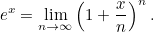(1)
If we consider the Taylor series for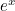, on the left hand side we have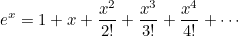On the right, we can expand out the product using the Binomial Theorem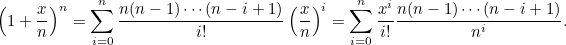The coefficient of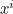equals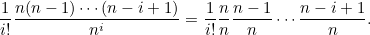As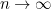each term here containing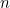converges togivingor exactly the Taylor series for.

You might think this argument has made somewhat cavalier use of limits. The following argument, adapted from [1, pg 272], is close to Euler's original. He was even more bold in his use of limits!

"Euler unhesitatingly accepts the existence of both infinitely small and infinitely large numbers, and uses them to such effect that the modern reader's own hesitation must be tinged with envy." [1, pg 272]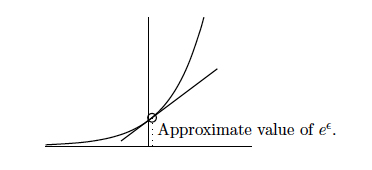Thinking aboutnear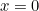, and its gradient, we see that, for infinitely small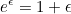This is illustrated above. Let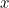be any given number, then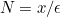is infinitely large. So

### Reference

 C.H. Edwards, The Historical Development of the Calculus, Springer-Verlag, 1979.